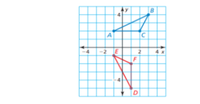# Hey guys, I need help deciding which transformation maps angle ABC to angle DEF

#### tannerr

##### New memberA. Rotation 90 degree counterclockwise around the origin. Then translation (x,y) -> (x+4, y-3)

B. Translation (x,y) -> (x-4, y-3) than 90 degree counterclockwise rotation around the origin.

C. Translation (x,y) -> (x+4, y-3) than 90 degree counter-clockwise rotation around the origin

D. Rotation 90 degrees counter-clockwise around the origin than translated to (x,y) -> (x-4, y-3)

#### Dr.Peterson

##### Elite Member
View attachment 27716
A. Rotation 90 degree counterclockwise around the origin. Then translation (x,y) -> (x+4, y-3)

B. Translation (x,y) -> (x-4, y-3) than 90 degree counterclockwise rotation around the origin.

C. Translation (x,y) -> (x+4, y-3) than 90 degree counter-clockwise rotation around the origin

D. Rotation 90 degrees counter-clockwise around the origin than translated to (x,y) -> (x-4, y-3)
Can you sketch the result of each transformation? You might first just see where each one takes B, and if more than one takes B to E, check the rest.

Let's see what you've done.

•topsquark